Open in App
Not now

# Python Program to Delete Specific Line from File

• Last Updated : 26 Sep, 2021

In this article, we are going to see how to delete the specific lines from a file using Python

Throughout this program, as an example, we will use a text file named months.txt on which various deletion operations would be performed.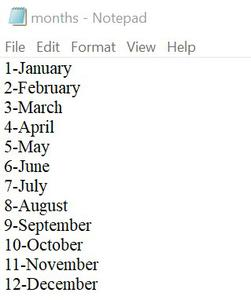## Method 1: Deleting a line using a specific position

In this method, the text file is read line by line using readlines(). If a line has a position similar to the position to be deleted, it is not written in the newly created text file.

Example:

## Python3

 `# deleting a line``# based on the position` `# opening the file in``# reading mode` `try``:``    ``with ``open``(``'months.txt'``, ``'r'``) as fr:``        ``# reading line by line``        ``lines ``=` `fr.readlines()``        ` `        ``# pointer for position``        ``ptr ``=` `1``    ` `        ``# opening in writing mode``        ``with ``open``(``'months.txt'``, ``'w'``) as fw:``            ``for` `line ``in` `lines:``              ` `                ``# we want to remove 5th line``                ``if` `ptr !``=` `5``:``                    ``fw.write(line)``                ``ptr ``+``=` `1``    ``print``(``"Deleted"``)``    ` `except``:``    ``print``(``"Oops! something error"``)`

Output:

`Deleted`

‘5-May’ was written on the 5th line which has been removed as shown below: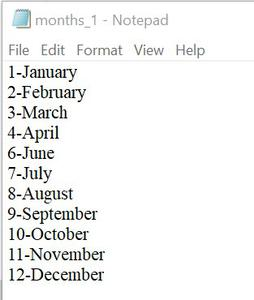## Method 2: Deleting a line using a text that matches exactly with the line

In this method, after reading the file, each line is checked if it matches with the given text exactly. If it does not match, then it is written in a new file.

## Python3

 `# deleting a line on the basis``# of a matching text (exactly)` `# we want to remove a line``# with text = '8-August'``try``:``    ``with ``open``(``'months.txt'``, ``'r'``) as fr:``        ``lines ``=` `fr.readlines()` `        ``with ``open``(``'months_2.txt'``, ``'w'``) as fw:``            ``for` `line ``in` `lines:``              ` `                ``# strip() is used to remove '\n'``                ``# present at the end of each line``                ``if` `line.strip(``'\n'``) !``=` `'8-August'``:``                    ``fw.write(line)``    ``print``(``"Deleted"``)``except``:``    ``print``(``"Oops! something error"``)`

Output:

`Deleted`

The line having ‘8-August’ exactly has been removed as shown: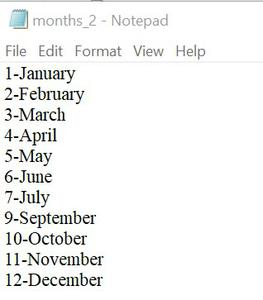## Method 3: Using custom-made logics

### Example 1: Deleting lines containing a specified pattern

Here, the lines containing the specified string pattern are removed. The pattern may not be necessarily the whole exact line.

## Python3

 `# deleting a line matching``# a specific pattern or``# containing a specific string` `# we want to delete a line``# containing string = 'ber'``try``:``    ``with ``open``(``'months.txt'``, ``'r'``) as fr:``        ``lines ``=` `fr.readlines()` `        ``with ``open``(``'months_3.txt'``, ``'w'``) as fw:``            ``for` `line ``in` `lines:``              ` `                ``# find() returns -1``                ``# if no match found``                ``if` `line.find(``'ber'``) ``=``=` `-``1``:``                    ``fw.write(line)``    ``print``(``"Deleted"``)``except``:``    ``print``(``"Oops! something error"``)`

Output:

`Deleted`

All the lines containing the pattern ‘ber’ such as ‘9-September’, ’10-October’, ’11-November’, ’12-December’ have been removed.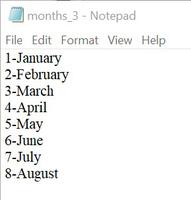### Example 2: Deleting lines with the condition

If we have a condition that the lines in our file must have a minimum length. So, the following example shows how to delete lines not having a minimum specified length.

## Python3

 `# deleting all the lines that are``# not having the minimum length``# excluding the newline '\n' character` `# let the min_len = 7``try``:``    ``with ``open``(``'months.txt'``, ``'r'``) as fr:``        ``lines ``=` `fr.readlines()` `        ``min_len ``=` `7` `        ``with ``open``(``'months_4.txt'``, ``'w'``) as fw:``            ``for` `line ``in` `lines:``                ``if` `len``(line.strip(``'\n'``)) >``=` `min_len:``                    ``fw.write(line)` `    ``print``(``"Deleted"``)``except``:``    ``print``(``"Oops! something error"``)`

Output:

`Deleted`

All the lines not having a length of greater than or equal to 7 have been removed: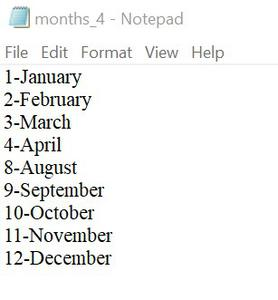My Personal Notes arrow_drop_up